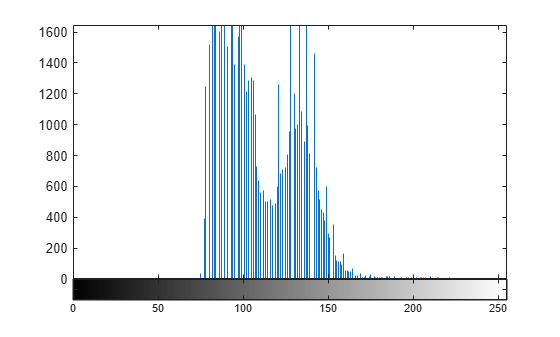# imhist

Histogram of image data

## Syntax

``````[counts,binLocations] = imhist(I)``````
``[counts,binLocations] = imhist(I,n)``
``[counts,binLocations] = imhist(X,cmap)``
``imhist(___)``

## Description

``````[counts,binLocations] = imhist(I)``` calculates the histogram for the grayscale image `I`. The `imhist` function returns the histogram counts in `counts` and the bin locations in `binLocations`. The number of bins in the histogram is determined by the image type.```
````[counts,binLocations] = imhist(I,n)` specifies the number of bins, `n`, used to calculate the histogram.```
````[counts,binLocations] = imhist(X,cmap)` calculates the histogram for the indexed image `X` with colormap `cmap`. The histogram has one bin for each entry in the colormap.```

example

````imhist(___)` displays a plot of the histogram. If the input image is an indexed image, then the histogram shows the distribution of pixel values above a color bar of the colormap `cmap`.```

## Examples

collapse all

Read a grayscale image into the workspace.

`I = imread('pout.tif');`

Display a histogram of the image. Since `I` is grayscale, by default the histogram will have 256 bins.

`imhist(I)``load mristack`

Display the histogram of the data. Since the image is grayscale, `imhist` uses 256 bins by default.

`imhist(mristack)`## Input Arguments

collapse all

Grayscale image, specified as a numeric array of any dimension. If the image has data type `single` or `double`, then values must be in the range [0, 1]. If `I` has values outside the range [0, 1], then you can use the `rescale` function to rescale values to the expected range.

Data Types: `single` | `double` | `int8` | `int16` | `int32` | `uint8` | `uint16` | `uint32` | `logical`

Number of bins, specified as a positive integer. If `I` is a grayscale image, then `imhist` uses a default value of 256 bins. If `I` is a binary image, then `imhist` uses two bins.

Example: `50`

Data Types: `single` | `double` | `int8` | `int16` | `int32` | `int64` | `uint8` | `uint16` | `uint32` | `uint64`

Indexed image, specified as a numeric array of any dimension.

Data Types: `single` | `double` | `uint8` | `uint16` | `logical`

Colormap associated with indexed image `X`, specified as a c-by-3 numeric matrix with values in the range [0, 1]. Each row is a three-element RGB triplet that specifies the red, green, and blue components of a single color of the colormap.The colormap must be at least as long as the largest index in `X`.

Data Types: `double`

## Output Arguments

collapse all

Histogram counts, returned as a numeric array. If the histogram is computed for an indexed image, `X`, then the length of `counts` is the same as the length of the colormap, `cmap`.

Bin locations, returned as a numeric array.

## Tips

• For grayscale images, the `n` bins of the histogram are each half-open intervals of width A/(n−1). In particular, the pth bin is the half-open interval

`$\frac{A\left(p-1.5\right)}{\left(n-1\right)}-B\le x<\frac{A\left(p-0.5\right)}{\left(n-1\right)}-B,$`

where x is the intensity value. The scale factor A and offset B depend on the type of the image class as follows:

Data TypeAB
`double``1``0`
`single``1``0`
`int8``255``128`
`int16``65,535``32,768`
`int32``4,294,967,295``2,147,483,648`
`uint8``255``0`
`uint16``65,535``0`
`uint32``4,294,967,295``0`
`logical``1``0`

• To display the histogram from `counts` and `binLocations`, use the command `stem(binLocations,counts)`.

## Version History

Introduced before R2006a

expand all